# 5.1.7 Free Body Diagrams

Higher Tier Only

### Examples of Forces

#### Tension

• Tension is:

The force experienced by a cable, rope, or string when pulled, hung, rotated or supported

• This is normally labelled as T on free body diagrams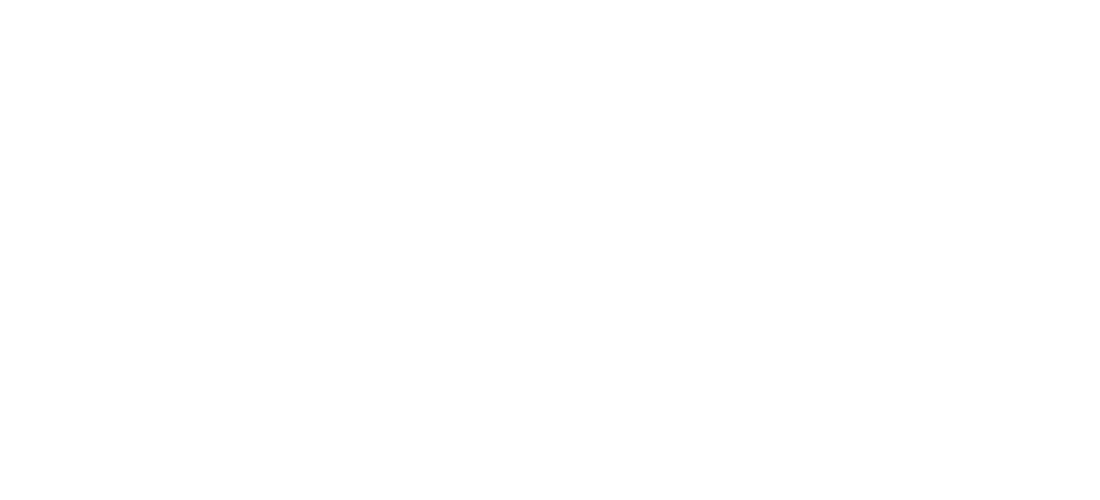Tension always acts away from the mass

#### Normal Contact Force

• The normal contact force is:

The force arising when an object rests against another object acting at a 90° angle to the plane of contact

• It is sometimes also referred to as the reaction force
• This is normally labelled as N or R on free body diagrams
• This force arises from Newton’s Third Law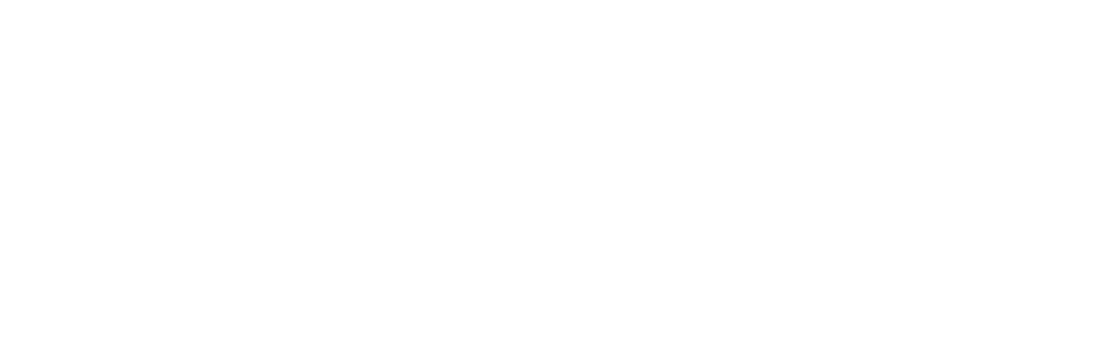Normal contact force always acts perpendicular to the surface

#### Upthrust

• Upthrust is:

The upward buoyancy force acting on an object when it is in a fluid

• Upthrust can occur in liquids and gases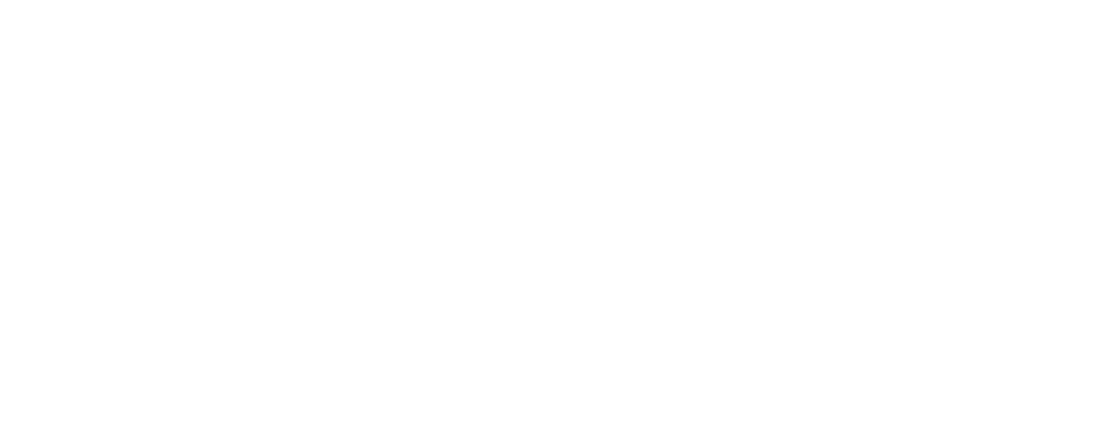Upthrust always acts upwards

#### Friction

• Friction is:

The force that arises when two surfaces are in contact with each other

• Friction always opposes the motion
• This is normally labelled as F or Fr on free body diagrams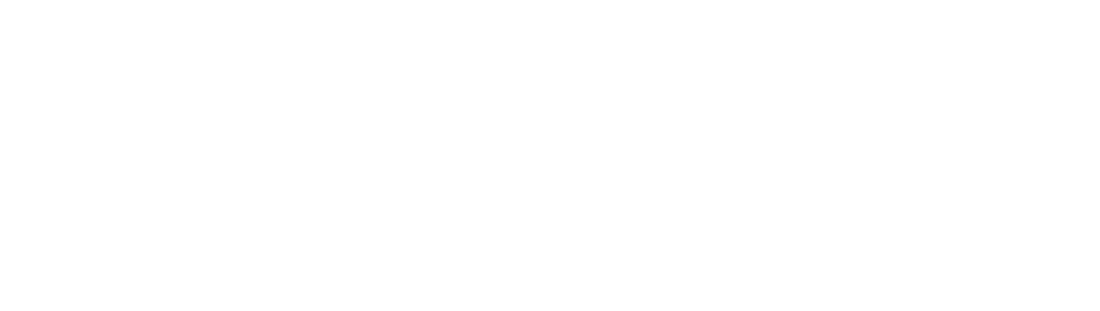Friction always acts at the point where the objects are in contact, and in the opposite direction to the direction of motion

Higher Tier Only

### Free Body Diagrams

• Free body diagrams are useful for modelling the forces that are acting on an object
• Each force is represented as a vector arrow, where each arrow:
• Is scaled to the magnitude of the force it represents
• Points in the direction that the force acts
• Is labelled with the name of the force it represents
• Free body diagrams can be used:
• To identify which forces act in which plane
• To resolve the net force in a particular direction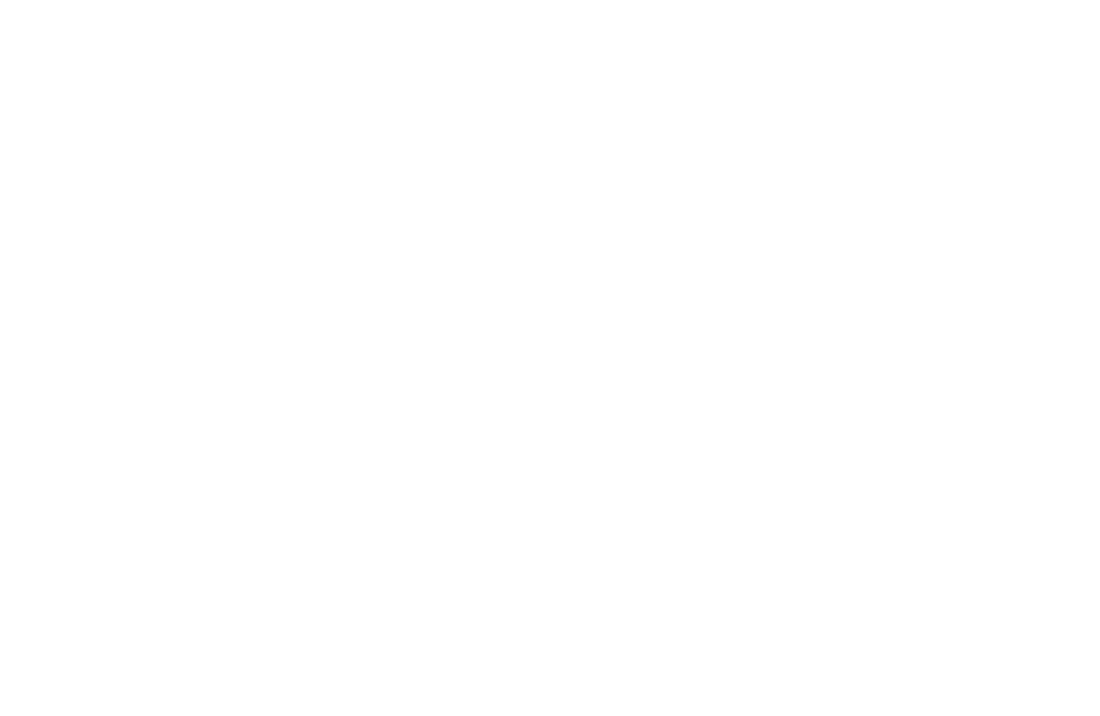Free body diagrams can be used to show the various forces acting on objects

#### Worked Example

Draw free-body diagrams for the following scenarios:

a) A picture frame hanging from a nail

b) A box sliding down a slope

c) A man fishing in a stationary boat

d) A car accelerating along a road

Part (a)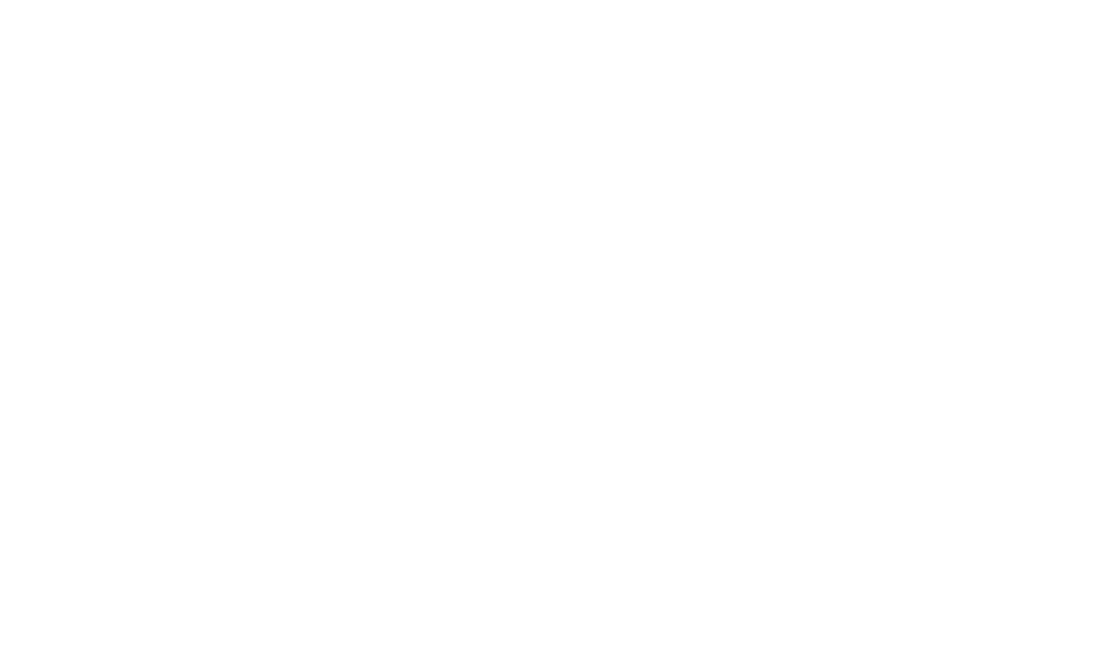• The size of the arrows should be such that the 3 forces would make a closed triangle as they are balanced

Part (b)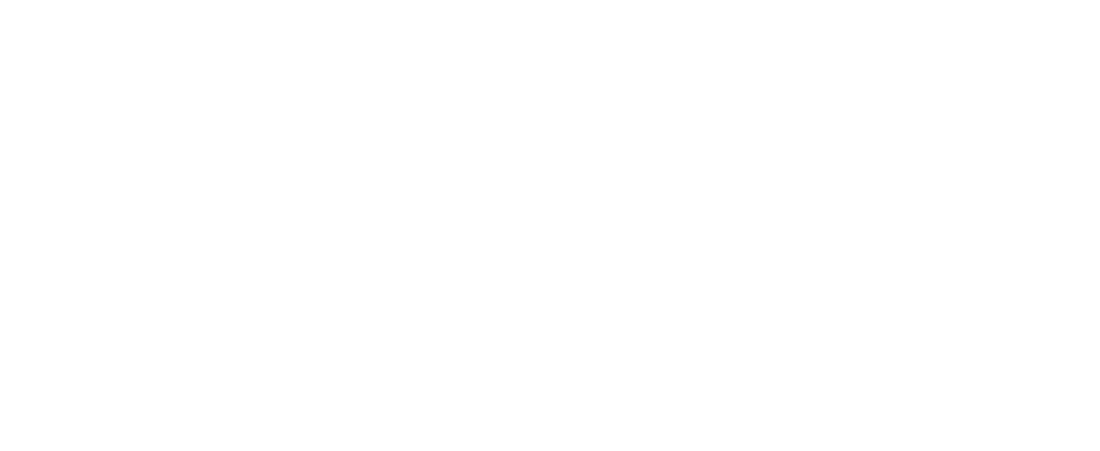• There are three forces acting on the box
• The normal contact force, R, acts perpendicular to the slope
• Friction, F, acts parallel to the slope and in the opposite direction to the direction of motion
• Weight, W, acts down towards the Earth

Part (c)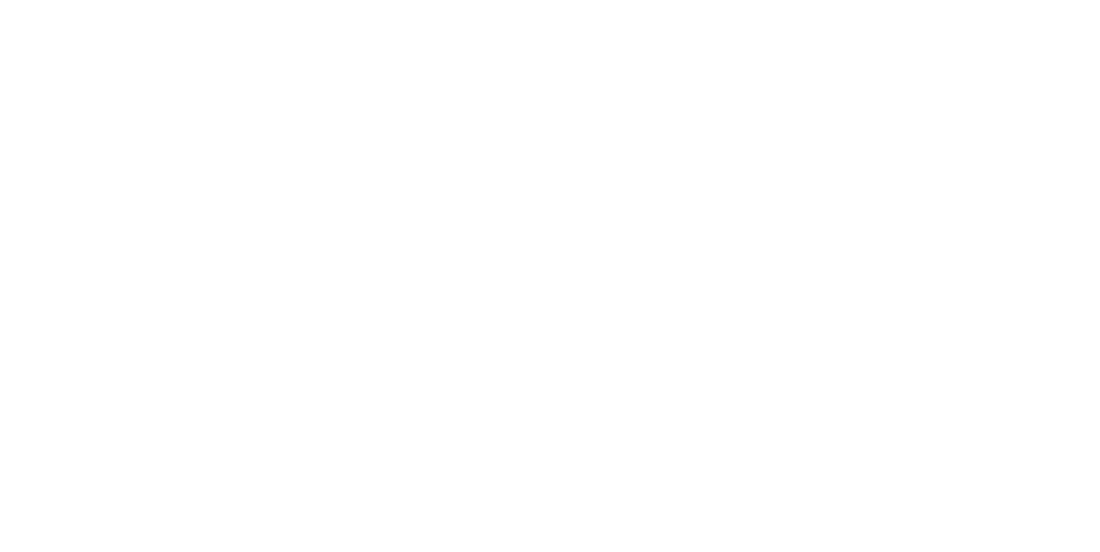• As the boat is not moving, the size of both arrows must be the same

Part (d)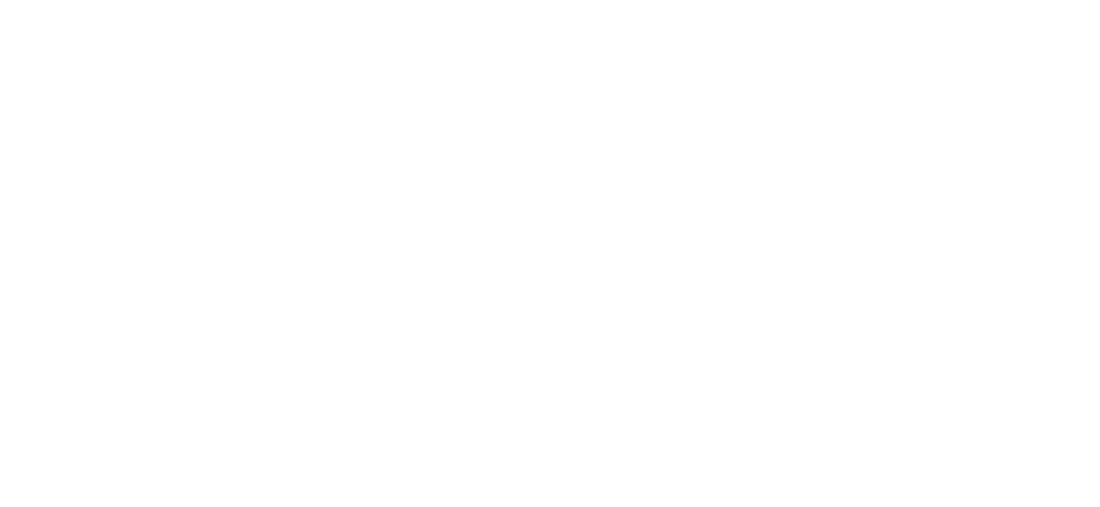• As the car is accelerating, the size of the thrust must be larger than the size of the friction force
• As in part (c), the upwards and downwards forces must be equal

#### Worked Example

Draw a free-body diagram of an toy sail boat with weight 30N floating in water that is being pulled to the right by an applied force of 35N.

Step 1: Draw the object in a simplified diagram

Step 2: Identify all of the forces acting upon the object in the question, including any forces that may be implied

• Weight: 30N down
• Upthrust from the water (since the object is floating): 30N up
• Applied force: 35N to the right

Step 3: Draw in all of the force vectors (arrows), making sure the arrows start at the object and are directed away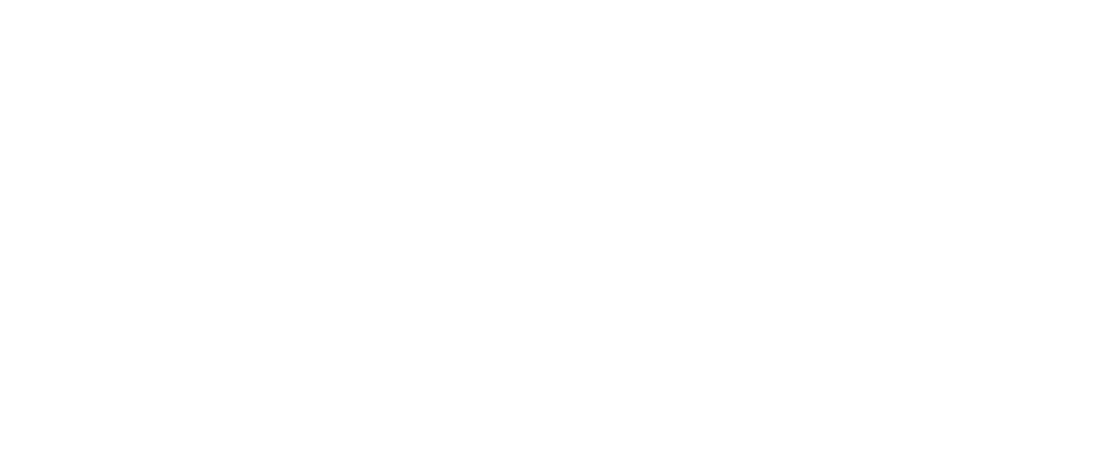• An approximation can be made as to the final resultant force due to all of the forces
• Decide whether the resultant force is approximately up or down
• Decide whether the resultant force is approximately left or right
• For example: The resultant force is directed up and to the right### Author: Katie

Katie has always been passionate about the sciences, and completed a degree in Astrophysics at Sheffield University. She decided that she wanted to inspire other young people, so moved to Bristol to complete a PGCE in Secondary Science. She particularly loves creating fun and absorbing materials to help students achieve their exam potential.
CloseClose